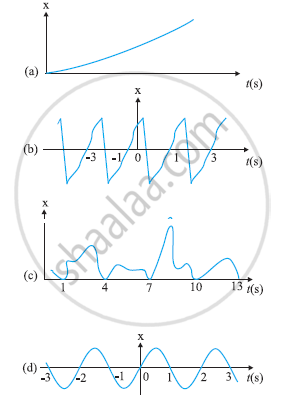# Figure Depicts Four X-t Plots for Linear Motion of a Particle. Which of the Plots Represent Periodic Motion? What is the Period of Motion (In Case of Periodic Motion) - Physics

Figure depicts four x-t plots for linear motion of a particle. Which of the plots represent periodic motion? What is the period of motion (in case of periodic motion)?#### Solution 1

(b) and (d) are periodic

(a) It is not a periodic motion. This represents a unidirectional, linear uniform motion. There is no repetition of motion in this case.

(b) In this case, the motion of the particle repeats itself after 2 s. Hence, it is a periodic motion, having a period of 2 s.

(c) It is not a periodic motion. This is because the particle repeats the motion in one position only. For a periodic motion, the entire motion of the particle must be repeated in equal intervals of time.

(d) In this case, the motion of the particle repeats itself after 2 s. Hence, it is a periodic motion, having a period of 2 s

#### Solution 2

Figure (b) and (d) represent periodic motions and the time period of each of these is 2 seconds, (a) and (c) are non-periodic motions.

Concept: Periodic and Oscillatory Motion
Is there an error in this question or solution?

#### APPEARS IN

NCERT Class 11 Physics
Chapter 14 Oscillations
Exercises | Q 3 | Page 357

Share